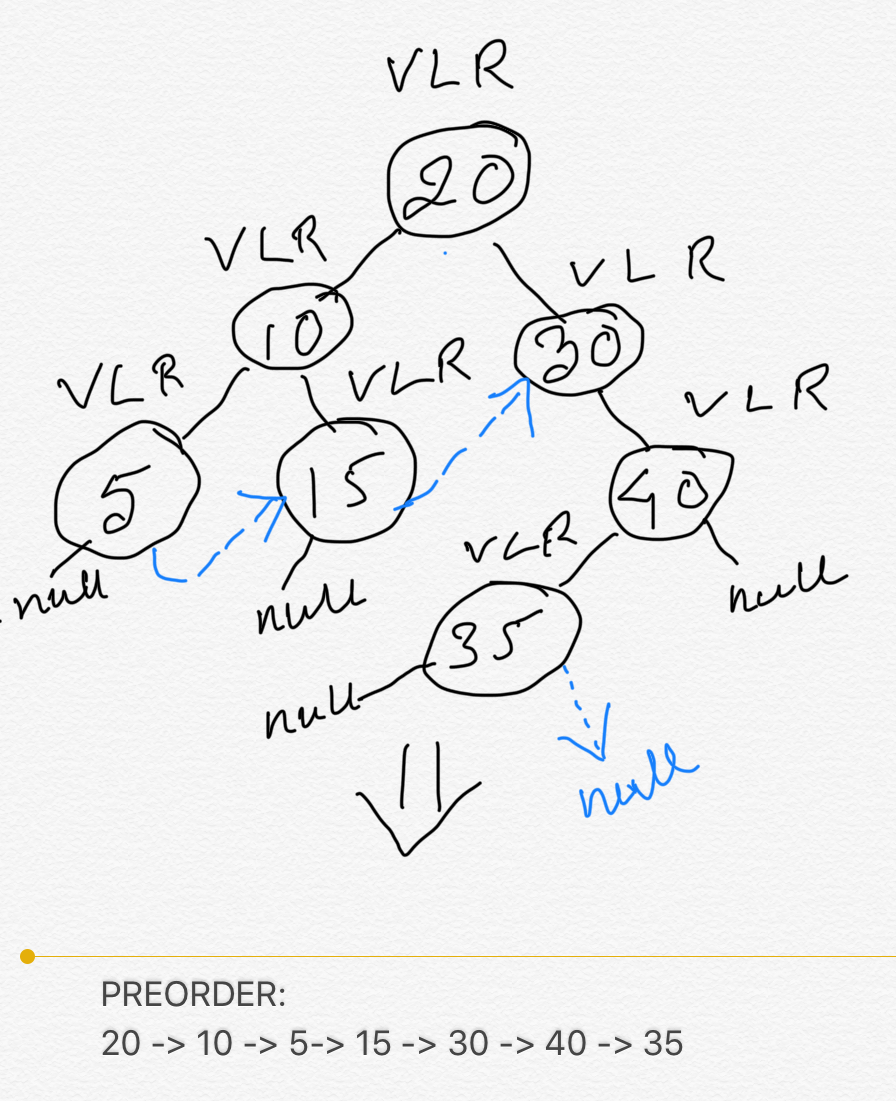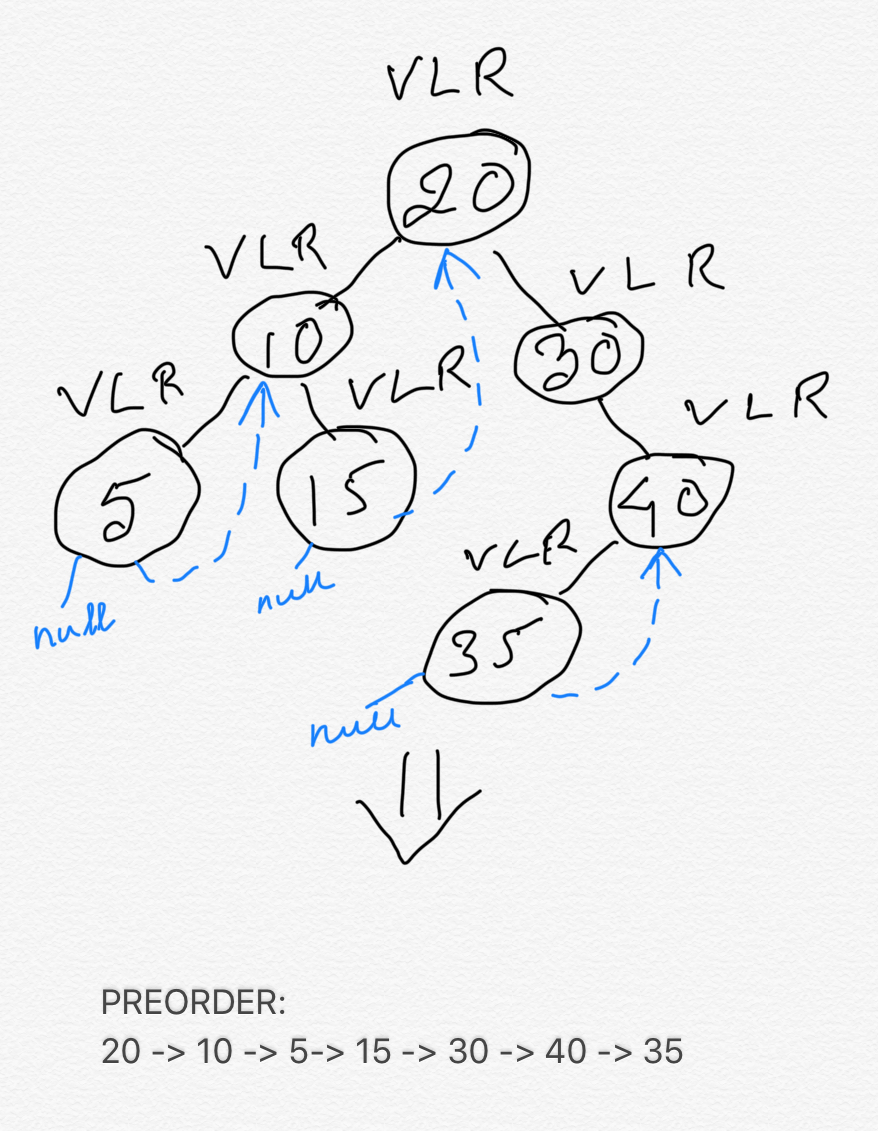#### Prerequisites:

I would highly recommend you to read the prerequisite chapters mentioned above first, if you haven't done so, to have a better and easy understanding of the contents discussed in this chapter.

Iterative (without using concept of Threaded Binary Tree) and Recursive approach of Binary Tree Traversal takes O(n) space in the worst case scenario when the tree is skewed or chained. Morris Preorder Traversal reduces the space complexity to O(1) by using the concept of Threaded Binary Tree which states that use the null left and/or right child pointer of the leaf nodes to add extra information to optimize space complexity.

In Preorder Traversal we visit the current node, then do Preorder traversal of its entire left subtree, followed by the Preorder Traversal of its entire right subtree. In traditional way of doing iterative (using stack) and recursive Preorder Traversal we need O(n) space in worst case scenario when Binary Tree is chained or skewed, and O(height of the binary tree) = O(log2n) when Binary Tree is balanced, where n = total number of nodes in the binary tree. It is because after processing each leaf node we need to backtrack to the right child of its inorder successor (the "next" node of this node in an inorder traversal). But what if the right child pointers of the leaf nodes (leaf nodes have both left and right child pointer as null) already point to the right child of the leaf node's inorder successor ? Would we still need to keep a stack ? the answer is no. Because we can directly jump to the "next" node by using the threaded link, i.e, using the right child pointer of each node (except the right most leaf of the right subtree of the node, because it would be the last node visited in Preorder Traversal and its next node would be null) , which was previously null, to now directly point to its next node.
The below diagram will help you understand the concept. The blue threaded links are the modified right child pointers of the leaf nodes to point to the next node.#### Java code:

``````
/**
* Definition for a binary tree node.
* public class TreeNode {
*     int val;
*     TreeNode left;
*     TreeNode right;
*     TreeNode() {}
*     TreeNode(int val) { this.val = val; }
*     TreeNode(int val, TreeNode left, TreeNode right) {
*         this.val = val;
*         this.left = left;
*         this.right = right;
*     }
* }
*/
class Solution {
public List<Integer> preorderTraversal(TreeNode root) {
List<Integer> res = new ArrayList<>();
TreeNode curr = root;
while (curr != null) {

if (curr.left != null) {
TreeNode node1 = getRightMostNodeOfLeftSubtree(curr);
node1.right = curr.right;
curr = curr.left;
} else {
curr = curr.right;
}
}
return res;
}

private TreeNode getRightMostNodeOfLeftSubtree(TreeNode node) {
TreeNode curr = node.left;
while (curr.right != null) {
curr = curr.right;
}
return curr;
}
}
```
```

#### Python code:

``````
# Definition for a binary tree node.
# class TreeNode(object):
#     def __init__(self, val=0, left=None, right=None):
#         self.val = val
#         self.left = left
#         self.right = right
class Solution(object):
# :type root: Node
# :rtype: List[int]
def preorderTraversal(self, root):
res = []
curr = root
while(curr != None):
res.append(curr.val)
if curr.left != None:
node1 = self.getRightMostNodeOfLeftSubtree(curr)
node1.right = curr.right
curr = curr.left
else:
curr = curr.right
return res

def getRightMostNodeOfLeftSubtree(self, node):
curr = node.left
while curr.right != None:
curr = curr.right
return curr

``````

### Further Optimization:

The above implementation has two major problems:
1. First of all, it does not closely adhere to the definition of the Threaded Binary Tree. In single threaded binary tree the threaded links point to inorder successor. In the above implementation, the threaded links point to the right child of the inorder successor.

I won't say it's a major problem though, it's kind of minor. We can always tweak a concept to solve a problem.
2. The second problem is a real major problem: we are modifying the right child pointer of the leaf nodes but never recovering them. This results in side-effects which are not desired in a Clean Code, unless and until we are explicitly told that side-effect is fine as per the given requirements. we are told that

We take care of these two problems by visiting each node twice (except the leaf nodes).
The right child pointers of the leaf nodes now do NOT point to the next node (successor node), but instead point to its inorder successor. So any non-leaf nodes are visited twice: since the right child pointers of leaf nodes are now pointing to the leaf node's inorder successor, the inorder successor is visited for the second time after the predecessor leaf node is visited. But this second visit proves to be highly beneficial: we take this second visit as an opportunity to RECOVER the modified right child pointer of the predecessor leaf node and change the right child of the leaf node to point back to null. After this is done, we just move on to the right child of the current node, which is the preorder successor of the leaf node.
The below diagram explains this:#### Space Complexity:

O(1), no extra space for traversal.

#### Time Complexity:

O(2n) i.e, O(n). Note in this solution we are visiting the non-leaf nodes twice, which is NOT the case in the first solution.

#### Instructor:### Abhishek Dey

#### Microsoft | University of Florida